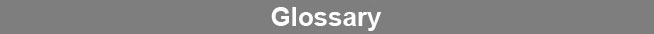Select Page### Expected Variance

Expected variance is the response variance expected at a single response point. This is computed from a variance regression derived from the means and variances of a pool of reference assay responses. Principle elements that factor in the variance regression are the heteroscedasticity of the test method, the random error, and the systematic error.www.brendan.com